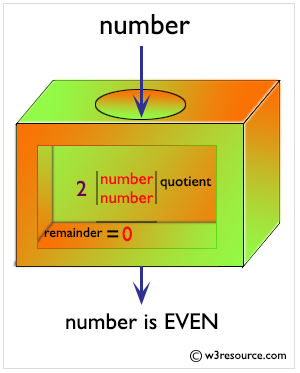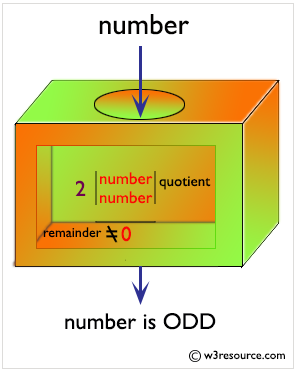# Python: Find whether a given number is even or odd, print out an appropriate message to the user

## Python Basic: Exercise-21 with Solution

Write a Python program to find whether a given number (accept from the user) is even or odd, print out an appropriate message to the user.
Pictorial Presentation of Even Numbers:Pictorial Presentation of Odd Numbers:Sample Solution:-
num = int(input("Enter a number: "))
mod = num % 2
if mod > 0:
print("This is an odd number.")
else:
print("This is an even number.")

========= RESTART: F:/Python_APSC/py-ex-basic-21.py ===========
Enter a number: 12
This is an even number.
>>>
========= RESTART: F:/Python_APSC/py-ex-basic-21.py ===========
Enter a number: 9
This is an odd number.
>>>